Vibrations and Waves - Lesson 0 - Vibrations

# Motion of a Mass on a Spring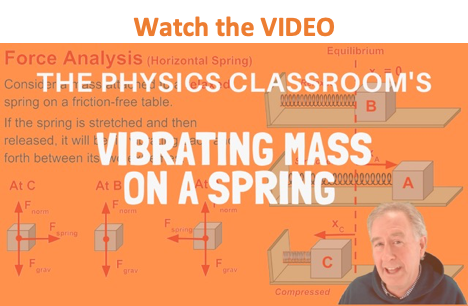In a previous part of this lesson, the motion of a mass attached to a spring was described as an example of a vibrating system. The mass on a spring motion was discussed in more detail as we sought to understand the mathematical properties of objects that are in periodic motion. Now we will investigate the motion of a mass on a spring in even greater detail as we focus on how a variety of quantities change over the course of time. Such quantities will include forces, position, velocity and energy - both kinetic and potential energy.

###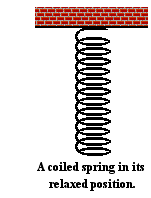Hooke's Law

We will begin our discussion with an investigation of the forces exerted by a spring on a hanging mass. Consider the system shown at the right with a spring attached to a support. The spring hangs in a relaxed, unstretched position. If you were to hold the bottom of the spring and pull downward, the spring would stretch. If you were to pull with just a little force, the spring would stretch just a little bit. And if you were to pull with a much greater force, the spring would stretch a much greater extent. Exactly what is the quantitative relationship between the amount of pulling force and the amount of stretch?

To determine this quantitative relationship between the amount of force and the amount of stretch, objects of known mass could be attached to the spring. For each object which is added, the amount of stretch could be measured. The force which is applied in each instance would be the weight of the object. A regression analysis of the force-stretch data could be performed in order to determine the quantitative relationship between the force and the amount of stretch. The data table below shows some representative data for such an experiment.

 Mass (kg) Force on Spring (N) Amount of Stretch (m) 0.000 0.000 0.0000 0.050 0.490 0.0021 0.100 0.980 0.0040 0.150 1.470 0.0063 0.200 1.960 0.0081 0.250 2.450 0.0099 0.300 2.940 0.0123 0.400 3.920 0.0160 0.500 4.900 0.0199

By plotting the force-stretch data and performing a linear regression analysis, the quantitative relationship or equation can be determined. The plot is shown below.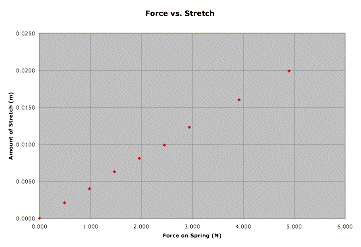A linear regression analysis yields the following statistics:

slope = 0.00406 m/N
y-intercept = 3.43 x10-5 (pert near close to 0.000)
regression constant = 0.999

The equation for this line is

Stretch = 0.00406•Force + 3.43x10-5The fact that the regression constant is very close to 1.000 indicates that there is a strong fit between the equation and the data points. This strong fit lends credibility to the results of the experiment.

This relationship between the force applied to a spring and the amount of stretch was first discovered in 1678 by English scientist Robert Hooke. As Hooke put it: Ut tensio, sic vis. Translated from Latin, this means "As the extension, so the force." In other words, the amount that the spring extends is proportional to the amount of force with which it pulls. If we had completed this study about 350 years ago (and if we knew some Latin), we would be famous! Today this quantitative relationship between force and stretch is referred to as Hooke's law and is often reported in textbooks as

Fspring = -k•x

where Fspring is the force exerted upon the spring, x is the amount that the spring stretches relative to its relaxed position, and k is the proportionality constant, often referred to as the spring constant. The spring constant is a positive constant whose value is dependent upon the spring which is being studied. A stiff spring would have a high spring constant. This is to say that it would take a relatively large amount of force to cause a little displacement. The units on the spring constant are Newton/meter (N/m). The negative sign in the above equation is an indication that the direction that the spring stretches is opposite the direction of the force which the spring exerts. For instance, when the spring was stretched below its relaxed position, x is downward. The spring responds to this stretching by exerting an upward force. The x and the F are in opposite directions. A final comment regarding this equation is that it works for a spring which is stretched vertically and for a spring is stretched horizontally (such as the one to be discussed below).

### Force Analysis of a Mass on a Spring

Earlier in this lesson we learned that an object that is vibrating is acted upon by a restoring force. The restoring force causes the vibrating object to slow down as it moves away from the equilibrium position and to speed up as it approaches the equilibrium position. It is this restoring force which is responsible for the vibration. So what is the restoring force for a mass on a spring?

We will begin our discussion of this question by considering the system in the diagram below.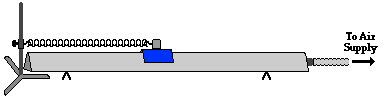The diagram shows an air track and a glider. The glider is attached by a spring to a vertical support. There is a negligible amount of friction between the glider and the air track. As such, there are three dominant forces acting upon the glider. These three forces are shown in the free-body diagram at the right. The force of gravity (Fgrav) is a rather predictable force - both in terms of its magnitude and its direction. The force of gravity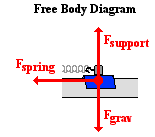always acts downward; its magnitude can be found as the product of mass and the acceleration of gravity (m•9.8 N/kg). The support force (Fsupport) balances the force of gravity. It is supplied by the air from the air track, causing the glider to levitate about the track's surface. The final force is the spring force (Fspring). As discussed above, the spring force varies in magnitude and in direction. Its magnitude can be found using Hooke's law. Its direction is always opposite the direction of stretch and towards the equilibrium position. As the air track glider does the back and forth, the spring force (Fspring) acts as the restoring force. It acts leftward on the glider when it is positioned to the right of the equilibrium position; and it acts rightward on the glider when it is positioned to the left of the equilibrium position.

Let's suppose that the glider is pulled to the right of the equilibrium position and released from rest. The diagram below shows the direction of the spring force at five different positions over the course of the glider's path. As the glider moves from position A (the release point) to position B and then to position C, the spring force acts leftward upon the leftward moving glider. As the glider approaches position C, the amount of stretch of the spring decreases and the spring force decreases, consistent with Hooke's Law. Despite this decrease in the spring force, there is still an acceleration caused by the restoring force for the entire span from position A to position C. At position C, the glider has reached its maximum speed. Once the glider passes to the left of position C, the spring force acts rightward. During this phase of the glider's cycle, the spring is being compressed. The further past position C that the glider moves, the greater the amount of compression and the greater the spring force. This spring force acts as a restoring force, slowing the glider down as it moves from position C to position D to position E. By the time the glider has reached position E, it has slowed down to a momentary rest position before changing its direction and heading back towards the equilibrium position. During the glider's motion from position E to position C, the amount that the spring is compressed decreases and the spring force decreases. There is still an acceleration for the entire distance from position E to position C. At position C, the glider has reached its maximum speed. Now the glider begins to move to the right of point C. As it does, the spring force acts leftward upon the rightward moving glider. This restoring force causes the glider to slow down during the entire path from position C to position D to position E.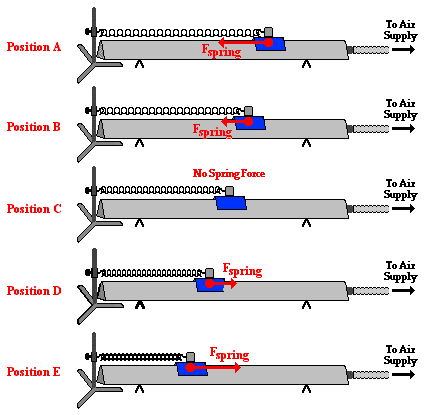### Sinusoidal Nature of the Motion of a Mass on a Spring

Previously in this lesson, the variations in the position of a mass on a spring with respect to time were discussed. At that time, it was shown that the position of a mass on a spring varies with the sine of the time. The discussion pertained to a mass that was vibrating up and down while suspended from the spring. The discussion would be just as applicable to our glider moving along the air track. If a motion detector were placed at the right end of the air track to collect data for a position vs. time plot, the plot would look like the plot below. Position A is the right-most position on the air track when the glider is closest to the detector.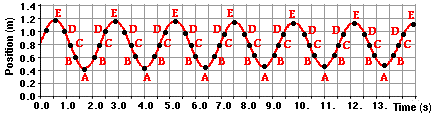The labeled positions in the diagram above are the same positions used in the discussion of restoring force above. You might recall from that discussion that positions A and E were positions at which the mass had a zero velocity. Position C was the equilibrium position and was the position of maximum speed. If the same motion detector that collected position-time data were used to collect velocity-time data, then the plotted data would look like the graph below.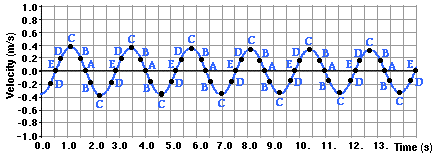Observe that the velocity-time plot for the mass on a spring is also a sinusoidal shaped plot. The only difference between the position-time and the velocity-time plots is that one is shifted one-fourth of a vibrational cycle away from the other. Also observe in the plots that the absolute value of the velocity is greatest at position C (corresponding to the equilibrium position). The velocity of any moving object, whether vibrating or not, is the speed with a direction. The magnitude of the velocity is the speed. The direction is often expressed as a positive or a negative sign. In some instances, the velocity has a negative direction (the glider is moving leftward) and its velocity is plotted below the time axis. In other cases, the velocity has a positive direction (the glider is moving rightward) and its velocity is plotted above the time axis. You will also notice that the velocity is zero whenever the position is at an extreme. This occurs at positions A and E when the glider is beginning to change direction. So just as in the case of pendulum motion, the speed is greatest when the displacement of the mass relative to its equilibrium position is the least. And the speed is least when the displacement of the mass relative to its equilibrium position is the greatest.

### Energy Analysis of a Mass on a Spring

On the previous page, an energy analysis for the vibration of a pendulum was discussed. Here we will conduct a similar analysis for the motion of a mass on a spring. In our discussion, we will refer to the motion of the frictionless glider on the air track that was introduced above. The glider will be pulled to the right of its equilibrium position and be released from rest (position A). As mentioned, the glider then accelerates towards position C (the equilibrium position). Once the glider passes the equilibrium position, it begins to slow down as the spring force pulls it backwards against its motion. By the time it has reached position E, the glider has slowed down to a momentary pause before changing directions and accelerating back towards position C. Once again, after the glider passes position C, it begins to slow down as it approaches position A. Once at position A, the cycle begins all over again ... and again ... and again.

The kinetic energy possessed by an object is the energy it possesses due to its motion. It is a quantity that depends upon both mass and speed. The equation that relates kinetic energy (KE) to mass (m) and speed (v) is

KE = ½•m•v2

The faster an object moves, the more kinetic energy that it will possess. We can combine this concept with the discussion above about how speed changes during the course of motion. This blending of the concepts would lead us to conclude that the kinetic energy of the mass on the spring increases as it approaches the equilibrium position; and it decreases as it moves away from the equilibrium position.

This information is summarized in the table below:

 Stage of Cycle Change in Speed Change in Kinetic Energy A to B to C Increasing Increasing C to D to E Decreasing Decreasing E to D to C Increasing Increasing C to B to A Decreasing Decreasing

Kinetic energy is only one form of mechanical energy. The other form is potential energy. Potential energy is the stored energy of position possessed by an object. The potential energy could be gravitational potential energy, in which case the position refers to the height above the ground. Or the potential energy could be elastic potential energy, in which case the position refers to the position of the mass on the spring relative to the equilibrium position. For our vibrating air track glider, there is no change in height. So the gravitational potential energy does not change. This form of potential energy is not of much interest in our analysis of the energy changes. There is however a change in the position of the mass relative to its equilibrium position. Every time the spring is compressed or stretched relative to its relaxed position, there is an increase in the elastic potential energy. The amount of elastic potential energy depends on the amount of stretch or compression of the spring. The equation that relates the amount of elastic potential energy (PEspring) to the amount of compression or stretch (x) is

PEspring = ½ • k•x2

where k is the spring constant (in N/m) and x is the distance that the spring is stretched or compressed relative to the relaxed, unstretched position.

When the air track glider is at its equilibrium position (position C), it is moving it's fastest (as discussed above). At this position, the value of x is 0 meter. So the amount of elastic potential energy (PEspring) is 0 Joules. This is the position where the potential energy is the least. When the glider is at position A, the spring is stretched the greatest distance and the elastic potential energy is a maximum. A similar statement can be made for position E. At position E, the spring is compressed the most and the elastic potential energy at this location is also a maximum. Since the spring stretches as much as compresses, the elastic potential energy at position A (the stretched position) is the same as at position E (the compressed position). At these two positions - A and E - the velocity is 0 m/s and the kinetic energy is 0 J. So just like the case of a vibrating pendulum, a vibrating mass on a spring has the greatest potential energy when it has the smallest kinetic energy. And it also has the smallest potential energy (position C) when it has the greatest kinetic energy. These principles are shown in the animation below.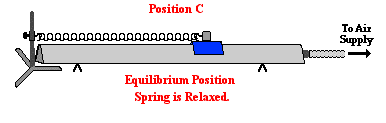When conducting an energy analysis, a common representation is an energy bar chart. An energy bar chart uses a bar graph to represent the relative amount and form of energy possessed by an object as it is moving. It is a useful conceptual tool for showing what form of energy is present and how it changes over the course of time. The diagram below is an energy bar chart for the air track glider and spring system.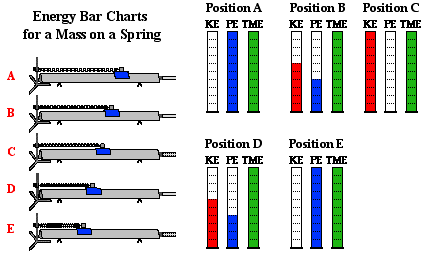The bar chart reveals that as the mass on the spring moves from A to B to C, the kinetic energy increases and the elastic potential energy decreases. Yet the total amount of these two forms of mechanical energy remains constant. Mechanical energy is being transformed from potential form to kinetic form; yet the total amount is being conserved. A similar conservation of energy phenomenon occurs as the mass moves from C to D to E. As the spring becomes compressed and the mass slows down, its kinetic energy is transformed into elastic potential energy. As this transformation occurs, the total amount of mechanical energy is conserved. This very principle of energy conservation was explained in a previous chapter - the Energy chapter - of The Physics Classroom Tutorial.

### Period of a Mass on a Spring

As is likely obvious, not all springs are created equal. And not all spring-mass systems are created equal. One measurable quantity that can be used to distinguish one spring-mass system from another is the period. As discussed earlier in this lesson, the period is the time for a vibrating object to make one complete cycle of vibration. The variables that effect the period of a spring-mass system are the mass and the spring constant. The equation that relates these variables resembles the equation for the period of a pendulum. The equation is

T = 2•Π•(m/k).5

where T is the period, m is the mass of the object attached to the spring, and k is the spring constant of the spring. The equation can be interpreted to mean that more massive objects will vibrate with a longer period. Their greater inertia means that it takes more time to complete a cycle. And springs with a greater spring constant (stiffer springs) have a smaller period; masses attached to these springs take less time to complete a cycle. Their greater spring constant means they exert stronger restoring forces upon the attached mass. This greater force reduces the length of time to complete one cycle of vibration.

### Looking Forward to Lesson 2

As we have seen in this lesson, vibrating objects are wiggling in place. They oscillate back and forth about a fixed position. A simple pendulum and a mass on a spring are classic examples of such vibrating motion. Though not evident by simple observation, the use of motion detectors reveals that the vibrations of these objects have a sinusoidal nature. There is a subtle wave-like behavior associated with the manner in which the position and the velocity vary with respect to time. In the next lesson, we will investigate waves. As we will soon find out, if a mass on a spring is a wiggle in time, then a wave is a collection of wigglers spread through space. As we begin our study of waves in Lesson 2, concepts of frequency, wavelength and amplitude will remain important.

### We Would Like to Suggest ...Why just read about it and when you could be interacting with it? Interact - that's exactly what you do when you use one of The Physics Classroom's Interactives. We would like to suggest that you combine the reading of this page with the use of our Mass on a Spring Interactive. You can find it in the Physics Interactives section of our website. The Mass on a Spring Interactive provides the learner with a simple environment for exploring the effect of mass, spring constant and duration of motion upon the period and amplitude of a vertically-vibrating mass.

1. A force of 16 N is required to stretch a spring a distance of 40 cm from its rest position. What force (in Newtons) is required to stretch the same spring …

a. … twice the distance?
b. … three times the distance?
c. … one-half the distance?

2. Perpetually disturbed by the habit of the backyard squirrels to raid his bird feeders, Mr. H decides to use a little physics for better living. His current plot involves equipping his bird feeder with a spring system that stretches and oscillates when the mass of a squirrel lands on the feeder. He wishes to have the highest amplitude of vibration that is possible. Should he use a spring with a large spring constant or a small spring constant?

3. Referring to the previous question. If Mr. H wishes to have his bird feeder (and attached squirrel) vibrate with the highest possible frequency, should he use a spring with a large spring constant or a small spring constant?

4. Use energy conservation to fill in the blanks in the following diagram.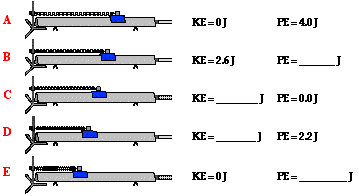5. Which of the following mass-spring systems will have the highest frequency of vibration?

Case A: A spring with a k=300 N/m and a mass of 200 g suspended from it.
Case B: A spring with a k=400 N/m and a mass of 200 g suspended from it.

6. Which of the following mass-spring systems will have the highest frequency of vibration?

Case A: A spring with a k=300 N/m and a mass of 200 g suspended from it.
Case B: A spring with a k=300 N/m and a mass of 100 g suspended from it.